Design of Experiments Guide > Taguchi Designs > Example of a Taguchi Design
Publication date: 08/13/2020

# Example of a Taguchi Design

The following example is an experiment described by Byrne and Taguchi (1986). The objective of the experiment is to find settings of control factors to maximize the adhesiveness (pull-off force) of nylon tubing. Four control factors are evaluated across three noise factors.

The signal factors for this example are as follows:

Interfer

Tubing and connector interference. Signal factor with 3 levels.

Wall

Wall thickness of the connector. Signal factor with 3 levels.

Depth

Insertion depth of the tubing into the connector. Signal factor with 3 levels.

Percent of adhesive. Signal factor with 3 levels.

The noise factors for this example are as follows:

Time

Conditioning time. Noise factor with 2 levels.

Temperature

Temperature. Noise factor with 2 levels.

Humidity

Relative humidity. Noise factor with 2 levels.

#### Create the Design

1. Select DOE > Classical > Taguchi Arrays.

2. Select Help > Sample Data Library and open Design Experiment/Byrne Taguchi Factors.jmp.

3. In the Taguchi Arrays window, click the Taguchi Design red triangle and select Load Factors.

The Factors panel shows the four three-level control (signal) factors and three noise factors.

Note: The Goal selected for the response determines the SN Ratio formula that is added to your design data table.

4. Ensure that L9-Taguchi is selected for the inner array.

5. Click L8 for the outer array design.

Figure 14.2 Completed Taguchi Design Window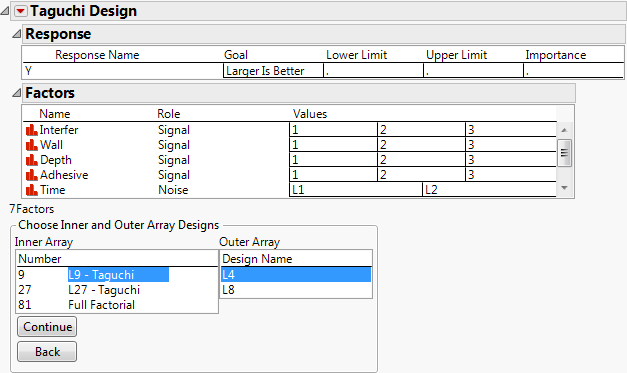6. Click Continue.

7. Click Make Table to create the design table shown in Figure 14.3.

The inner array has nine runs for the four signal factors, each at three levels. The settings for the signal factors are provided in the first four columns of the table.

The outer design is a full factorial design for the three two-level noise factors. The outer array is provided in eight columns of the data table. The column names are the pattern of the outer array runs. For example, the column named “---” is for the results collected when all of the noise levels are at their low levels. Each of the nine trials are conducted at each of the eight combination of noise factors for a total of 72 experimental trials.

Figure 14.3 Taguchi Design before Data Entry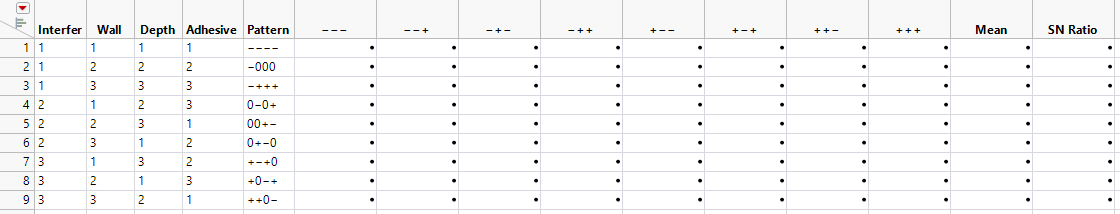The design table allows for entry of the 72 experimental results.

8. Select Help > Sample Data Library and open Design Experiment/Byrne Taguchi Data.jmp.

Figure 14.4 Complete Taguchi Design Table (Byrne Taguchi Data.jmp)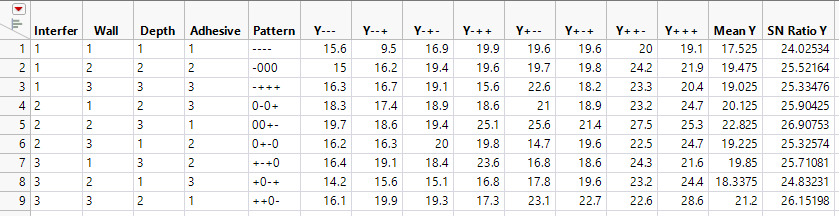The SN Ratio Y column is the performance statistic (larger is better) to maximize the response. It is computed as –10 times the common logarithm of the average of the squared reciprocals of the responses: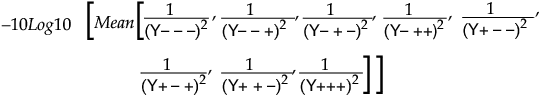This expression is large when all of the individual y response values are large. That is, you are trying to find the signal settings that result in the largest response across the noise settings.

#### Analyze the Data

The data are now ready to analyze. The goal of the analysis is to find factor settings that maximize both the mean and the signal-to-noise ratio.

1. In the Byrne Taguchi Data.jmp data table, click the green arrow to run the Model script.

Figure 14.5 Fit Model Launch Window for Taguchi Data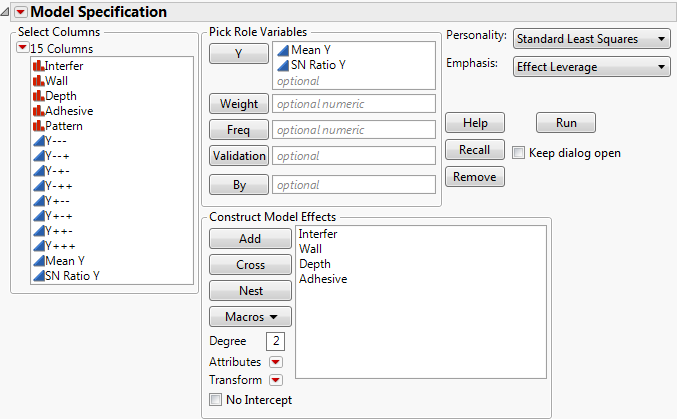The script launches the Fit Model window. The model includes the main effects of the four signal factors to model the mean (Mean Y) and signal-to-noise ratio (SN Ratio Y) responses.

2. Click Run.

The Prediction Profiler at the bottom of the report is a quick way to find settings that give the highest signal-to-noise ratio for this experiment and the mean value at that maximum.

Figure 14.6 The Prediction Profiler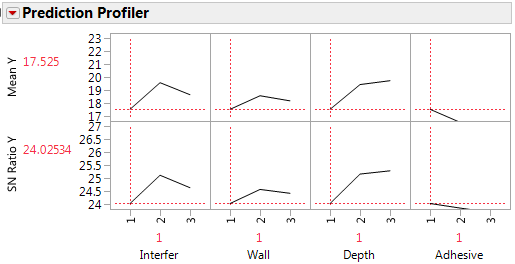3. Click the Prediction Profiler red triangle and select Optimization and Desirability > Desirability Functions.

This adds a row of traces and a column of function settings to the profiler, as shown in Figure 14.7. The default desirability functions are set to larger-is-better, which is what you want in this experiment. See Profiler in Profilers for more information about desirability functions in the prediction profiler.

4. Click the Prediction Profiler red triangle and select Optimization and Desirability > Maximize Desirability.

Figure 14.7 Best Factor Settings for Byrne Taguchi Data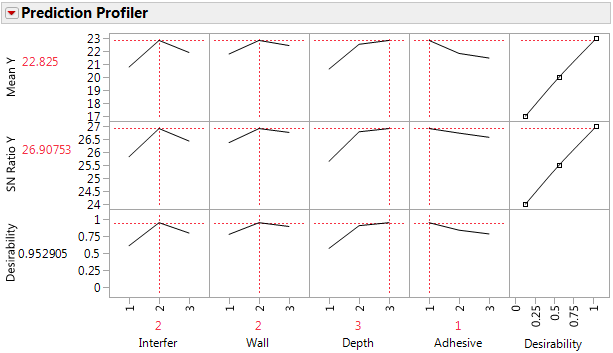In this example, the optimal settings for Interfer and Wall are 2, Depth is 3, and Adhesive is 1. These settings result in a predicted Mean value of 22.8 and an SN Ratio of 26.9.